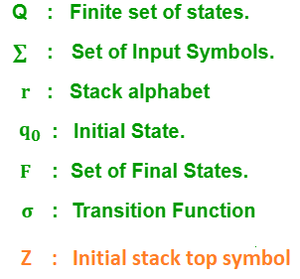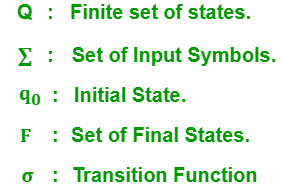Difference between Pushdown Automata and Finite Automata

• Last Updated : 30 Jun, 2021

Pushdown Automata:
A Pushdown automata (PDA) is a finite state machine with an added stack storage. Additional stack is used in making the decision for transitions apart from input symbols and current state. It contains the following 7 tuples:Finite Automata:
A Finite Automata is a mathematical model of any machine by which we can calculate the transition of states on every input symbol.Each transition in finite automata depends on the input symbols and current transition state. It contains the following 5 tuples:Let’s see the difference between Pushdown Automata and Finite Automata:

My Personal Notes arrow_drop_up